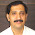### Current Transformer Application

Instrument Transformer and Power Management (P1) Course
Chapter (6) : Current Transformers
6.9 Current Transformer Application
We can use the current transformers in either measuring or protection, So we will study two examples on the C. T applications :
1-How to use C. Tin measuring.
2-How to use C. Tin protection.

6.9.1 How to use C.T in measuring:
Equipment required :
A.C supply 220 volts.                                            Resistive load 250 ohm.
Wattmeter instrument.                                            Current transformer
Procedure  :
1-Connect the circuit as shown.
2-From the turns ratio test:
NXl -X2 / NHl -H2 = I / 1
NXl -X3 / NHl -H2 = 2 / 1
When we connect the coil XI -X2 , P measuring = ( 190W).
When we connect the coil XI -X3 , P measuring = ( 95 W).
When we connect the coil XI -X4 , P measuring = ( 63 W).
Since, P actual = P measuring x CT turns ratio Then, we can complete the following table and so we find the actual power is the same in all cases.
 P actual P measuring Turns X1-X2 X1-X3 X1-X4

6.9.2 How to use C. T in protection :
Equipment protection :
A.C supply 220 volts.                                      Three phase over current relay.
Procedure  :
1-Connect the circuit as shown.
We find that Ir + Is + It = 0. This means that, the over current relay will not operate But at fault condition, say phase "R" is opened.
Then, the summation of current passing through the relay will not equal to zero and the relay will operate.

From the phasor diagram, the Victorian summation of It + Is = -Ir. When Ir = O the current passing through the relay will equal to Is + It. If two phases had opened say "R & S" , we find that the same current passes through the relay and the relay will operate.

1.